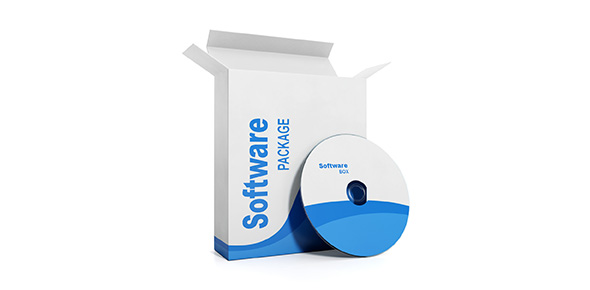# Advanced Python Test - 2

15 Questions | Attempts: 511SettingsIf you are a programmer then you must have some knowledge on Python. Are you just getting into programming are studying advanced python? This second quiz is designed to gauge your understanding of this language. Give it a try and see how much you score. Good luck!

• 1.
What does the following code do? def a(b, c, d): pass
• A.

Defines a list and initializes it

• B.

Defines a function, which does nothing

• C.

Defines a function, which passes its parameters through

• D.

Defines an empty class

• 2.
What gets printed? Assuming python version 2.x print type(1/2)
• A.

• B.

What gets printed? Assuming python version 2.x print type(1/2)

• C.

• D.

• E.

• 3.
What is the output of the following code? print type([1,2])
• A.

• B.

• C.

• D.

• E.

• 4.
What gets printed? def f(): pass print type(f())
• A.

• B.

• C.

• D.

• E.

• 5.
What should the below code print? print type(1J)
• A.

• B.

• C.

• D.

• E.

• 6.
What is the output of the following code? print type(lambda:None)
• A.

• B.

• C.

• D.

• E.

• 7.
What is the output of the below program? a = [1,2,3,None,(),[],] print len(a)
• A.

Syntax error

• B.

4

• C.

5

• D.

6

• E.

7

• 8.
What gets printed? Assuming python version 3.x print (type(1/2))
• A.

• B.

• C.

• D.

• E.

• 9.
What gets printed? d = lambda p: p * 2 t = lambda p: p * 3 x = 2 x = d(x) x = t(x) x = d(x) print x
• A.

7

• B.

12

• C.

24

• D.

36

• E.

48

• 10.
What gets printed? x = 4.5 y = 2 print x//y
• A.

2.0

• B.

2.25

• C.

9.0

• D.

20.25

• E.

21

• 11.
What gets printed? nums = set([1,1,2,3,3,3,4]) print len(nums)
• A.

1

• B.

2

• C.

4

• D.

5

• E.

7

• 12.
What gets printed? x = True y = False z = False   if x or y and z:     print "yes" else:     print "no"
• A.

Yes

• B.

No

• C.

Fails to compile

• 13.
What gets printed? x = True y = False z = False   if not x or y:     print 1 elif not x or not y and z:     print 2 elif not x or y or not y and x:     print 3 else:     print 4
• A.

1

• B.

2

• C.

3

• D.

4

• 14.
If PYTHONPATH is set in the environment, which directories are searched for modules? A) PYTHONPATH directory B) current directory C) home directory D) installation dependent default path
• A.

A only

• B.

A and D

• C.

A, B, and C

• D.

A, B, and D

• E.

A, B, C, and D

• 15.
In python 2.6 or earlier, the code will print error type 1 if accessSecureSystem raises an exception of either AccessError type or SecurityError type try:   accessSecureSystem() except AccessError, SecurityError:   print "error type 1"   continueWork()
• A.

True

• B.

FalseBack to top
×

Wait!
Here's an interesting quiz for you.